Karlström, A., Hill, J., and Johansson, L. (2023). “Data-driven soft sensors in refining processes – Pulp property estimation using ARX – models,” BioResources 18(4), 8163-8186.

#### Abstract

This paper focuses on estimation of shives(wide) and fiber length in RGP82CD-refiners using an AutoRegressive eXogenous (ARX) structure in a data-driven soft sensor concept. Both external and internal variables are considered as model inputs. The pulp properties were sampled every 15 min from an on-line device positioned after the latency chest, whereas other process data were sampled every 6 seconds. Notably, despite the high data sampling rate, the development of robust models necessitated a dataset spanning over two months of process information. The external variables studied in this paper were specific energy, the sawmill chip content, plate gaps, and dilution water feed rates to each refining zone. Additional internal variables, such as the inlet flat zone temperature, the maximum temperature, and the periphery temperature in the conical zone, were also used as model inputs. It was concluded that both shives(wide) and fiber length can be estimated with relatively good accuracy although large uncertainties exist in the measured properties. Finally, it was shown that fast pulp property dynamics in the blow-line can be followed, which outperforms current practices of using pulp measurement devices positioned after the latency chest. This offers implementation of more advanced future pulp property control concepts.

Data-Driven Soft Sensors in Refining Processes – Pulp Property Estimation Using ARX – Models

Anders Karlström,a Jan Hill,b and Lars Johansson c,*

This paper focuses on estimation of shives(wide) and fiber length in RGP82CD-refiners using an AutoRegressive eXogenous (ARX) structure in a data-driven soft sensor concept. Both external and internal variables are considered as model inputs. The pulp properties were sampled every 15 min from an on-line device positioned after the latency chest, whereas other process data were sampled every 6 seconds. Notably, despite the high data sampling rate, the development of robust models necessitated a dataset spanning over two months of process information. The external variables studied in this paper were specific energy, the sawmill chip content, plate gaps, and dilution water feed rates to each refining zone. Additional internal variables, such as the inlet flat zone temperature, the maximum temperature, and the periphery temperature in the conical zone, were also used as model inputs. It was concluded that both shives(wide) and fiber length can be estimated with relatively good accuracy although large uncertainties exist in the measured properties. Finally, it was shown that fast pulp property dynamics in the blow-line can be followed, which outperforms current practices of using pulp measurement devices positioned after the latency chest. This offers implementation of more advanced future pulp property control concepts.

DOI: 10.15376/biores.18.4.8163-8186

Keywords: Soft sensors; Consistency; Temperature profile; Pulp property estimations; CTMP; ARX models

Contact information: a: Chalmers University of Technology, Department of Electrical Engineering, SE-412 96 Gothenburg, Sweden; b: QualTech Jan Hill AB, SE-282 21 Tyringe, Sweden; c: Woodworks Cluster, Skognæringa Kyst, Skolegata 22, Landbruksklyngen bygg P2, NO-7713 Steinkjer, Norway;

* Corresponding author: lars@woodworkscluster.no

INTRODUCTION

In the last two decades, soft sensors have been established as valuable complements to traditional measurements for critical process variables, process monitoring, and process control. The term soft sensors imply the usage of software, usually computer programs. The soft sensors, based on the models, are expected to deliver similar information as their hardware counterparts (if they exist). In the process industry, such predictive sensors are also called inferential sensors, see e.g., Jordaan et al. (2004) and Qin (1997), virtual online analyzers, see Han and Lee (2002), and observer-based sensors (Goodwin 2000).

Two different classes of soft sensors are often discussed in the literature: model-driven and data-driven soft sensors. The model-driven family is most commonly based on first principle models (FPM), extended Kalman filter, and adaptive observers (Bastin and Dochain 1990; Chruy 1997; Assis and Filho 2000).

In refining processes, related to mechanical pulping, FPMs have been developed over many years (Miles and May 1990, 1991; Huhtanen 2004). Originally, quite complex computational fluid dynamic models were in focus in describing the fiber, water, and steam distribution along the radius of the refining segments (Karlström et al. 2008). Although such models are not suitable to implement in on-line applications, Karlström and Eriksson (2014a,b,c,d) show that a reduced 2-dimensional non-linear model can be derived for temperature and consistency control applications (Karlström and Hill 2018b,c).

When it comes to the development of data-driven soft sensors, some popular modelling techniques for principal component analysis, system identification, and artificial neural networks are quite powerful (Ljung 1997; Principe et al. 2000; Jolliffe 2002; Wold et al. 2001). Such techniques are useful when studying refining processes (Berg 2005; Eriksson 2005, 2009). The literature describing refining processes is comprehensive (Hill et al. 1979, 1993; Johansson et al. 1980; Dahlqvist and Ferrari 1981; Oksum 1983; Honkasalo et al. 1989; Bengtsson et al. 2021).

In this paper the authors combine the two different classes:

1) Model-driven soft sensors (“white-box” models – phenomenological knowledge about the process background (Karlström et al. 2008; Karlström and Eriksson 2014a,b,c,d); and

2) Data-driven soft sensors (black-box models – based on empirical observations of the process (Karlström et al. 2015b, 2016a,b; Karlström and Hill 2017a,b,c, 2018b; Karlström et al. 2018a,d).

There are many combinations of the two classes often referred to as hybrid models. In this paper they are referred to as grey-box models according to the terminology presented by Bohlin (2006).

As described by Karlström et al. (2017a,b,c), both external variables (specific energy, dilution water feed rates, plate gaps, and amount of sawmill chips) and internal variables (temperature profile, refining zone consistencies, and fiber residence time, etc.) can be considered as predictors when modeling pulp properties in commercial full-scale CTMP (Chemi Thermo Mechanical Pulping) production lines. The problem, however, is the measurement accuracy in the pulp devices. This is nothing new, as noted by Ferritius et al. (2018) in another format “the assumption that coarseness is constant for all particles, as well as the arithmetic average, may lead to erroneous conclusions in real life as well as in simulations when used as a measure of the number of long fibres”. In the absence of other more reliable pulp property measurements, the measured shives(wide) and fiber length provided by the pulp device are still used in this article, which calls for analysis of a huge amount of data.

One important issue discussed by Bengtsson et al. (2021), was that the use of internal and/or external variables in serially linked refining zones that can introduce collinearities. This is not a problem if a model-driven soft sensor model is available on-line, as the estimated internal variables are changed simultaneously when the external variables are changed. However, when changing specific external variables (such as plate gaps or dilution waters added to each refining zone) in data-driven soft sensors, other external variables (such as specific energy) are also affected. This prevents the use of the data-driven soft sensor as a standalone estimator. The idea presented in this article is to highlight this conceptual dilemma and start to analyze both external and internal variables as predictors. It is of special interest to consider the motor load split between the two refining zones in the CD-refiner in terms of the specific energy related to the flat zone (FZ) and the conical zone (CD), respectively. The temperature profiles in the FZ and CD as predictors will also be analyzed. To estimate shives(wide) and fiber length, the data-driven dynamic soft sensors derived in this article are based on a time-invariant linear system approach and specifically an AutoRegressive eXogenous (ARX) structure (Ljung 1997).

EXPERIMENTAL

CD-82 Refiner

This section covers the fundamental issues in how complex data sets from a full-scale CTMP production line can be used when modeling pulp and handsheet properties. The production line consists of an RGP82CD (Valmet, Sundsvall, Sweden) with three small 6 MW refiners (RLP54) in parallel. The CD refiner consists of two serially linked refining zones called the flat zone (FZ) and the conical zone (CD), see Fig. 1. In each zone, sensor arrays with eight temperature sensors have been mounted to measure the entire temperature profiles (Karlström and Hill 2017a, 2017b, 2017c).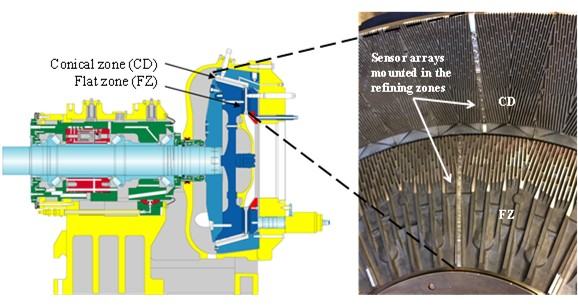Fig. 1. A schematic drawing of a CD82 refiner. The vertical flat zone (FZ) is directly linked to the conical zone (CD) via an expanding point.

Each temperature in Fig. 1 can be seen as an internal variable that is measured together with traditional process variables (external variables), such as production rate, dilution water flows, motor load, and plate gaps, between refining segments. These process variables constitute the inputs to a physical non-linear model, which the authors call the model-driven soft sensor, which is schematically described in Fig. 2. The model-driven soft sensor was developed to combine information about the fast dynamics in the refining zone (ranging from 0.5 to 1 s) with the slower actuator dynamics for plate gap changes and the overall dominant dynamics in the inlet and outlet piping. When running such models in real time, it is important to describe the dynamics at different production levels with varying process conditions to cope with the non-linearities in the process (Karlström and Eriksson 2014a,b,c,d) provide details). The model-driven soft sensor in Fig. 2 provides outputs that are controlled using TCtrl (maximum temperature control in FZ and CD) by manipulating the production and CCtrl (consistency control in FZ and CD) where the dilution water feed rates are controlled to each refining zone (Karlström et al. 2022). As can be seen in Fig. 2, the motor load split is available from the non-linear model, and this makes it possible to control the specific energy separately in each refining zone. That option has not been used so far, but in future applications two new concepts will be highlighted (Fig. 2), viz. ECtrl (specific energy control in FZ and CD) and QCtrl (quality control out from the CD-refiner), which constitutes the means in future research activities to derive a complete refining soft sensor concept.

The purpose of this paper was to develop a data-driven soft sensor that estimates the pulp properties shives(wide) and fiber length. Of course, the set of pulp properties can be expanded to include most of the fractions achieved from pulp sampling devices (positioned after the latency chest). However, this article focuses on a subset of all possible input candidates (predictors).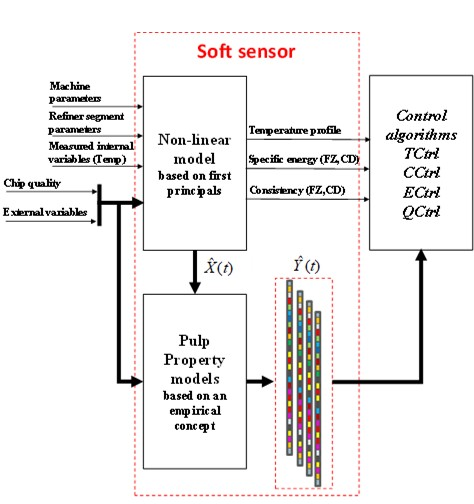Fig. 2. The soft sensor concept includes one block for the non-linear model based on first principles (model-driven soft sensor) and one for the pulp property models (data-driven soft sensor). In this set up, the data-driven soft sensor is based on an ARX-structure.

Input Candidates

Together, the model-driven soft sensor and the data-driven soft sensor constitute a “grey-box modeling” concept according to Bohlin (2006). In process modelling, knowledge of the process under consideration is typically partial with significant unknown inputs (disturbances) to the model. Disturbances militate against the desirable trait of model reproducibility. “Grey-box” identification can assist, in these circumstances, by taking advantage of the two sources of information that may be available: any invariant prior knowledge and response data from experiments.” Several inputs must be considered, especially the input candidates to the data-driven soft sensors.

As indicated in Fig. 2, the model-driven soft sensor provides several predictors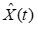, such as

• temperature profiles (> 20 variables);
• consistency profiles (> 20 variables);
• backwards and forward flowing steam (> 20 variables);
• water content profiles (> 20 variables);
• forces on bars (> 20 variables);
• defibration and thermodynamical work in each refining zone (> 4 variables).

These predictors can be used together with traditional external variables such as production and motor load. Karlström and Hill (2017a,b,c) provide a deeper analysis. If more than 100 input candidates are available as indicated above and five of them are chosen, it was formerly necessary to consider more than 75 million combinations (Bengtsson et al. 2019). This, of course, is a huge number of combinations to test and is impossible to handle practically. Therefore, only a fraction of all possible input candidates were analyzed.

As stated by Karlström et al. (2020), internal and external variables in serially linked refining zones can introduce (larger or smaller) collinearities between the inputs. This is a drawback for all data-driven soft sensors if the aim is to derive “pure” standalone estimators for pulp properties. This statement is most easily explained by an example: Consider a change in the plate gap in the FZ. This directly affects the specific energy, which most often is also an input in soft sensors.

To analyze and detect multicollinearities the Variance Inflation Factors (VIF) will be used, that is,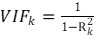(1)

where corresponds to the coefficient of determination obtained by regressing the kth inputs on the remaining inputs, i.e., VIF quantify how much the variance is inflated. A VIFk = 1 means that there is no linear correlation between the kth input and the other remaining inputs. If VIFk > 4, a general rule is that further analysis should be performed, while VIFk > 10 indicates serious multicollinearities, which may call for further analysis and perhaps a modified set of inputs (Belsley et al. 1980). In serially linked processes, such as the one studied in this article, the collinearities occur between some of the internal variables which means that several aspects must be analyzed. Further discussion is provided in the Appendix. In this article, the authors primarily focus on the external variables as they introduce fewer collinearities.

If the model-driven soft sensor is available, then data-driven soft sensors can be improved by including some linear independent internal variables. The most obvious external variables were focused; specific energy (Spe (kWh/T)), dilution water feed rate (dilFZ, dilCD (L/min)), plate gaps (gapFZ, gapCD (mm)), and amount of sawmill chips (ChipQ (%)). As internal variables, the initial temperature in the FZ (Tinit (degC)), the maximum temperature in the FZ (TmaxFZ (degC)), and the periphery temperature in the conical zone (TCDper (degC)) are included to minimize the risk of introducing collinearities between the predictors. When appropriate, two items are introduced: 1)SpeFZ and SpeCD, i.e., the specific energy related to each refining zone, and 2) the complete set of the most requested internal states such as the consistencies and fiber residence time in each zone beside two backward flowing steam velocities. The idea is to show how to use specific internal variables available from the motor-driven soft sensor.

Methodology

In terms of the data-driven soft sensor concept, a dynamic model approach based on primarily an AutoRegressive eXogenous (ARX) structure was used.

Consider the system description in Fig. 3 The input vector u(t) represents the external variables, such as production, the plate gaps and dilution water feed rates to the flat zone and conical zone, while vector x(t) represents the internal variables, such as refining zone temperatures, consistencies, and fiber residence times.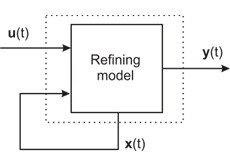Fig. 3. Illustration of modeling approaches: u(t) is the basic input vector, y(t) is the output vector, and x(t) is a vector of internal states

Hence, from a system identification perspective, the main input vector will be ux(t)={u(t),x(t)}. The focus is on a modeling and verification approach using AutoRegressive eXogenous (ARX) models, as outlined by Ljung (1999). The process output y(t) that is influenced by i different inputs, (u1x(t),u2x(t),… uix(t)), can be written in the following form,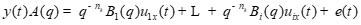(2)

where A and Bi are polynomials in the shift operator q and e(t) is a white noise term, i.e., a direct error in the difference equation. To fully understand Eq. 2, the delay operator is also included, as the dynamics (from u to y) sometimes contain a delay of nk samples. See Ljung (1999) for details. However, the sampling in u(t) is much faster compared with the sampling in y(t), which means that the delay can be ignored.

The ARX model in Eq. 2 describes both the system dynamics and noise properties using the same set of poles. The output y(t) corresponds to the pulp property vector described by, e.g., shives(wide) and fiber length.

All model parameters to be identified can be gathered in a column vector (see Eq. 3),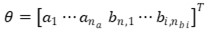(3)

where na, nb1,…, nbi are the order of the polynomials A, B1,…, Bn, respectively. In this case the polynomials A has the form: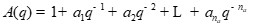(4)

The parameters of vector θ are determined using model prediction errors that are taken as the difference between the predicted outputs, often denoted ŷ, and the measured output sequence ym. These are sampled together with the inputs when acquiring data for the identification procedure. A prediction error norm, for example is Eq. 5,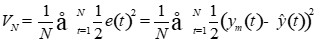(5)

will be minimized and, obviously, a small value of VN should correspond to a model with high accuracy in the predictions. As indicated in Fig. 3, the box labeled refining model includes both information from the flat zone and the conical zone when estimating the pulp properties.

Time Variant System

Large data sets are important when analyzing industrial unit operations. In this article, the process data from the refiner comes from two periods of more than A) 800 h for training the models and B) 1400 h used as a validation set. These time series are rather reliable from a measurement perspective and comprise a sampled period with few stops. The fiber residence time inside the refining zone is about one second. It is possible to follow this resolution by measuring the temperature profile inside the refining zones. The actuators can respond on a 30 s basis, which means that this dynamic sets the limit for how to design TCtrl and CCtrl. Nevertheless, all data sampled from the refining process in this article have a sampling rate of 6 s.

One obstacle, which is related to the pulp property measurement device in Fig. 4, is that the sampling rate is non-equidistant within an interval of 15 to 20 min. This causes uncertain sampling delays that must be considered in combination with the uncertainties in the measurement device itself. To overcome some of the problems, the signal can be resampled by using interpolation. This introduces, however, an estimate based on a assumed equidistant sampling rate. Therefore, an alternative is to use the ARMAX structure where the equation error is introduced as a moving average of white noise, i.e., instead of e(t) in Eq. 2, i.e.,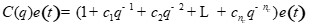(6)

is not an option, see further Ljung (1999).

An additional shortcoming, when using the measurement device after the latency chest in Fig. 4 to find proper models, is that the latency chest itself introduces an uncertainty.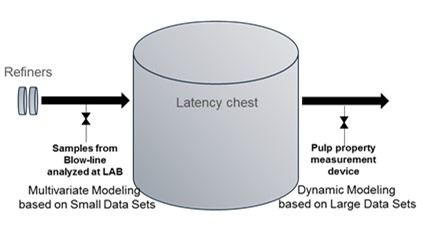Fig. 4. Schematic drawing, showing the sampling points for blow-line samples and the pulp property measurements after the latency chest

It can be seen as a time-variant system serially linked to the refiner and all these challenges introduce many uncertainties and we cannot expect to get a perfect model fit with this set up (Sund et al. 2021). However, known uncertainties must be suppressed. In this paper the method is straightforward. The idea is to reject all data where the outputs to be predicted are constant over a longer period than 2000 to 6000 samples, which corresponds to about 3 to 10 h. This precaution is taken as measuring equipment faults may occur during certain periods. The time series is divided into training and validation sets, the parameters are estimated using the training set, and some of the model alternatives are compared on the validation set using the coefficient of determination (R2):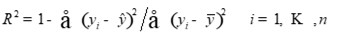(7)

Here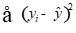represents the sum of the squared residuals from the regression while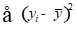denotes the sum of the squared differences from the mean of the dependent variable for n observations (Draper and Smith 1998).

Finally, because the goal is to derive an ARX model that describes the process before the latency chest, i.e., in the blow-line, the low-frequency gains (ki) obtained from the dynamic models,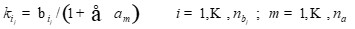(8)

will be used. Here, nb represents the number of predictors and j represents the pulp property studied while na corresponds to the size of the A-polynomial in Eq. 4.

Thereby, the latency chest dynamics can be suppressed. In other words, the dynamics in the latency chest as well as the dynamics in the measurement device and the noise sequence are ignored. This also makes it possible to compare the steady state gains with the parameters in the multivariate static models derived by Karlström and Hill (2017a,b,c).

RESULTS AND DISCUSSION

This section covers the results obtained when estimating pulp properties associated with the block describing the data-driven soft sensor in Fig. 2. The focus will be to derive soft sensors with a minimized set of linear independent predictors. As indicated in the Appendix, the multicollinearities, which are detected by using the Variance Inflation Factors (VIF) in Eq. 1, are minimized if the external variables are used as predictors. If internal variables are included as predictors, then it is important to verify low interdependencies between the predictors, as outlined in the Appendix. In this section it is shown that some internal variables are interesting to include as predictors. This does not mean that the model-driven soft sensor must be included in the model, as the temperature profile can be used without the non-linear model in Fig. 2. In contrast, if consistencies and/or for instance the specific energy split between FZ and CD will be used to improve the model accuracy, then internal variables derived from the model-driven soft sensor must be included as well.

There is motivation to use low-frequency gains in modeling: As concluded by Karlström et al. (2020), the model accuracy for freeness (i.e., the total volume of water discharged from a side orifice of a specific configuration while the pulp suspension drains freely under gravity) is unfortunately not good enough, as the measures need repeatable bias compensations after comparison with laboratory tests. This procedure does not ensure the accuracy in the measurement of the freeness. Although such changes occurred during the period described in Karlström et al. (2020), the estimation of freeness failed completely. This might be the most important contribution in this article series, because freeness is often used by operators as a measure for manual process control. Therefore, this paper focused on shives(wide) and fiber length as the main pulp properties described by Eq. 2. The methodology is outlined above and a deeper discussion of outlier rejection, etc. is given by Karlström et al. (2020).

Karlström et al. (2020) showed that the filtering of the estimates from the dynamic model was considerable because of the dynamics in the latency chest and “sluggish” sampling in the pulp measurement device corresponds to about 30 to 40 min. The fiber residence time is approximately one second in the refiner, while normal control actions, related to dynamics in actuators etc., correspond to time constants of about 2 to 3 min (Karlström et al. 2020). All this means that the sampling device after the latency chest is inappropriate for use for control actions. This is strengthened by studying Fig. 5, where the response from a dynamic model (red line) is far from the dynamics in the refiner itself.

Note that in all figures below, the detrended process values (mean values are removed) are shown instead of the actual measurements and estimates.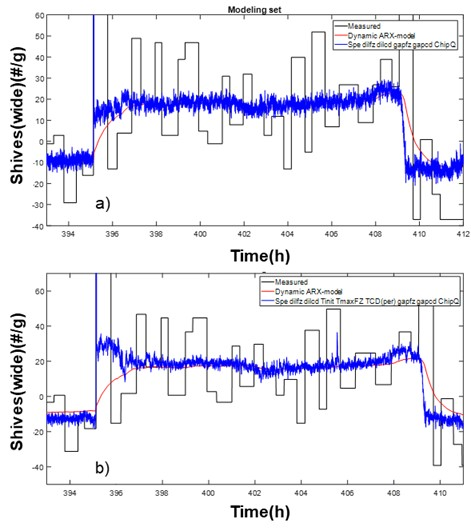Fig. 5. Close-up of a response in shives(wide) caused by changes in the production. The red line corresponds to the dynamic model responses (including dynamics in latency chest and measurement device). The blue line represents the static model response for two sets of predictors, see legend in a) and b). The black line represents the measured pulp property after the latency chest.

Through using the low-frequency gains (see response in Fig. 5a (blue line)), the time constant and the delays in the latency chest and measurement device can be ignored. A closer analysis indicates that direction-dependent dynamics were present. The settling time varied between 90 to 120 min. In the main text, the time constant is assumed to be 30 to 40 min, which is a conservative assumption motivated when the latency chest volume is less than 50% of its capacity. It is also important to mention that the consistency in the latency chest can vary, which of course affects the measurement accuracy as well. This is unfortunately often overlooked in the literature. It can be concluded that this introduces a tool for pulp quality control that compares the estimates with samples taken directly in the blow-line. It is also worth mentioning that additional predictors, in terms of internal variables in Fig. 5b, normally add information about overshoots in the responses.

Training Sets

The principles used when training models were outlined by Karlström et al. (2020), and here the focus is on the external inputs: specific energy (Spe), the amount of sawmill chips (ChipQ), plate gaps (gapFZ, gapCD), and dilution water added to each refining zone (dilFZ, dilCD). The internal variables, inlet temperature in FZ (Tinit), maximum temperature in FZ (TmaxFZ), periphery temperature in CD (TCD(per)), and the specific energy in the FZ and CD, will be studied as well.

Karlström et al. (2020) concluded that it is important to include the total specific energy (Spe) and the amount of sawmill chips (ChipQ) in all models for shives(wide) and fiber length. This assumption is used in this paper as well although this statement is not obvious when studying Table 1.

Table 1. Mean Square Error Ratios for Different Models for Shives(wide) and Fiber Length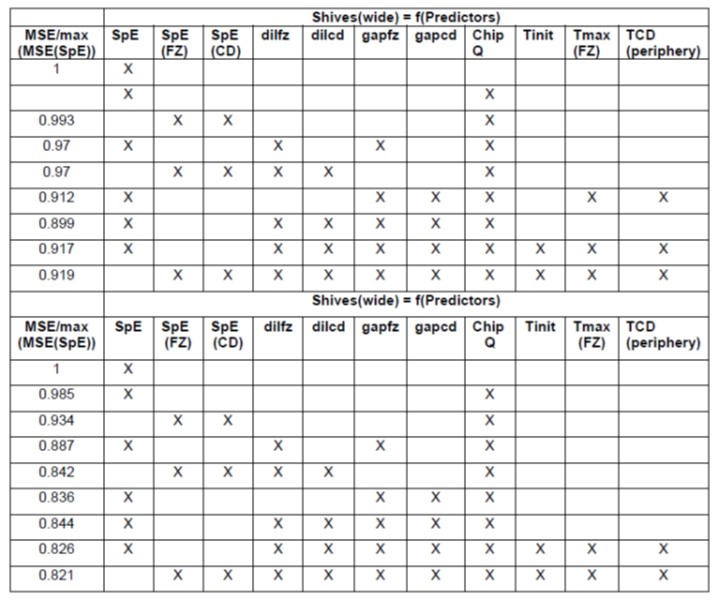As can be seen in Table 1, a complete analysis of the impact from each predictor was not performed in this paper, as the aim was to obtain a good enough model. Moreover, only minor improvements were obtained when the predictors SpE(FZ) and SpE(CD) were introduced instead of the total specific energy (SpE). However, this was true for this time series, where the external variables in the refining zone (such as dilution water feed rates and plate gaps in the FZ and CD) were not changed in the opposite direction or individually manipulated. Such situations can occur when the production is maximized or when the refining segments are not responding appropriately close to the end of the life cycle. To illustrate that, an extended time series of fiber length is shown, where the last 200 h before a plate change are included (Fig. 6).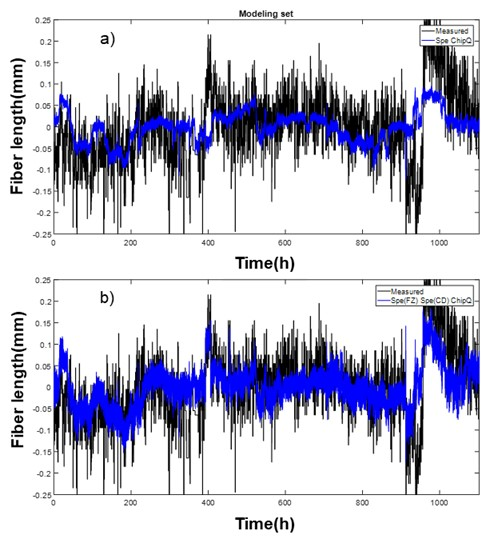Fig. 6. The measured and estimated fiber length using the low-frequency gains outlined in Eq. 8. a) Predictors used are specific energy and chip quality. b) Predictors used are specific energy in each refining zone and chip quality.

It is interesting to note that the same conclusion cannot be drawn by studying only shives(wide). If the plate gaps in the flat zone and conical zone are kept relatively stable over time this is a minor problem. Therefore, it is preferred to use the total specific energy when estimating both fiber length and shives(wide). Nevertheless, as seen in Table 1, the best fit when modeling shives(wide) was obtained when external variables were used as predictors while a mix of external and internal variables is desirable when estimating the fiber length. Other internal variables such as consistencies are not considered in this paper, as the dilution water and consistency are strongly correlated to each other.

Further model improvements seem to be difficult to obtain with this setup of predictors, and it can be concluded that the model responses in Fig. 7, at least, follow the trends in the time series.

The ARX-model parameters for the training set are given in Table 2 together with two new sets of ARX-model parameters for both shives(wide) and fiber length. The latter set was thereby used for comparison of both the original parameters and the validation sets.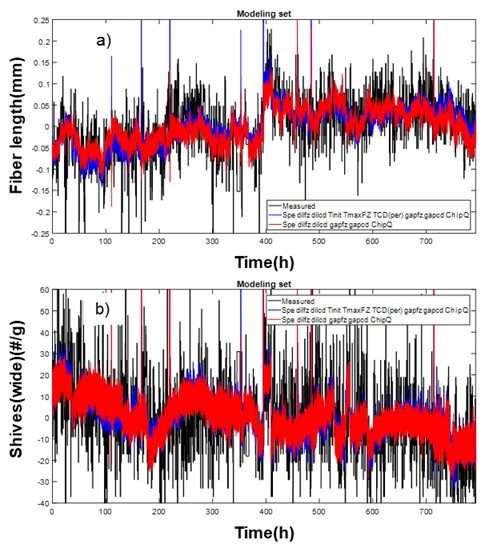Fig. 7. a) Measured and estimated fiber length using the low-frequency gains outlined in Eq. 8. Predictors used are given in the legend. b) Measured and estimated shives(wide) where the predictors are the same as in part b).

In general, any models like those in Table 2 are troublesome to use as standalone models due to interconnections between the predictors, see further the discussion in the appendix, and other issues that cause several challenges related to process non-linearities. As an example, consider the set of parameters in Case G, in Table 2.

In real life, a reduced plate gap in CD will most likely reduce the fiber length, while an increased plate gap can hardly increase the fiber length. This is often called direction-dependent dynamics, which is impossible to follow by only studying changes in the predictors. Hence, in this case the parameter sign is irrelevant, as it has no meaning in a “standalone”-perspective. At the same time, a reduced plate gap in CD will increase the specific energy, while an increased plate gap will reduce the specific energy. In other words, when it comes to specific energy dynamics, the process behavior can be described by a time-invariant linear system. In point, these two predictors are interconnected, which makes it difficult to use the model as a standalone model. Similar behavior occurs changing the dilution water feed rates are changed, resulting in a linear approximation of the non-linear process behavior. Therefore, it is important to state that the models in Table 2 do not necessarily give the best fit. Instead, the concept requires both a model- and data- driven concept to provide models that are good enough for the validation procedure. Note that the parameters shown in Table 2 correspond to the complete training set, i.e., 800 h. However, it is possible in some cases to limit the training set by using a cumulative method, where the time series is divided into n cumulative frames, where the parameter stability is analyzed in each frame (see Fig 8).

Table 2. Estimated Low-frequency Gain Parameters for Shives(wide) and Fiber Length*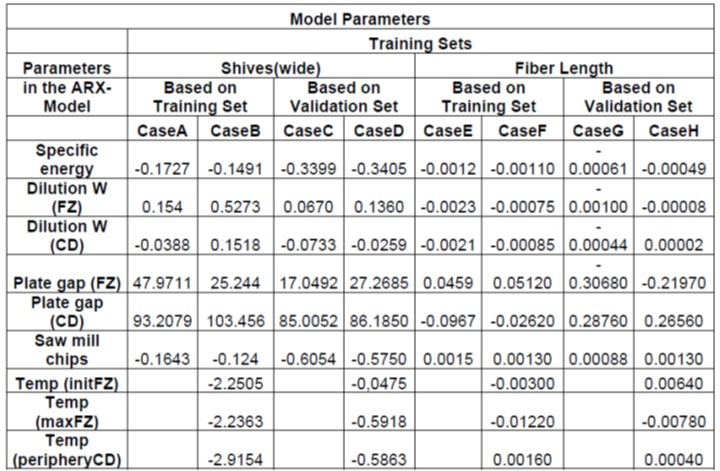*Based on the inputs: ux1 = [Spe, dilFZ, dilCD, gapFZ, gapCD, ChipQ] and ux2 = [Spe, dilFZ, dilCD, Tinit TmaxFZ TCD(per) gapFZ, gapCD, ChipQ], respectively

Some of the parameters seemed to converge when the number of frames reached 30, which corresponds to about 240 h while the parameters representing the dilution water feed rate are more cumbersome from a stability perspective.

When studying Fig. 7, it is obvious that both fiber length and shives(wide) had measurement uncertainties that can be cumbersome to include in a modeling procedure. To understand such uncertainties in the pulp property measurements, it is interesting to analyze R2 versus the window size of a moving average filter applied on model inputs and outputs (Ljung 1999). As can be seen in Fig. 9, a window size of about 4000 samples seemed to be the best for the two sets of predictors in Table 2, although the absolute values for the coefficient of determinations are questionable. It is noteworthy, however, that the introduction of internal states seemed to improve the prediction, as discussed below.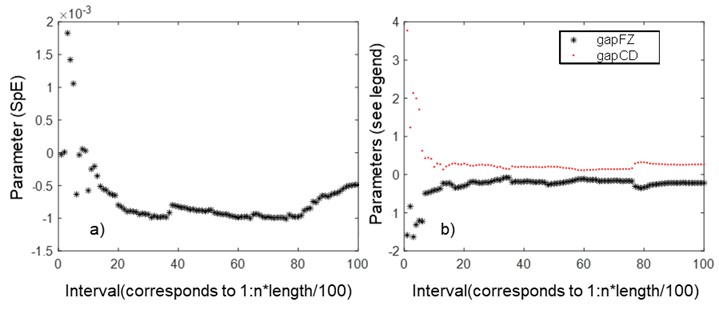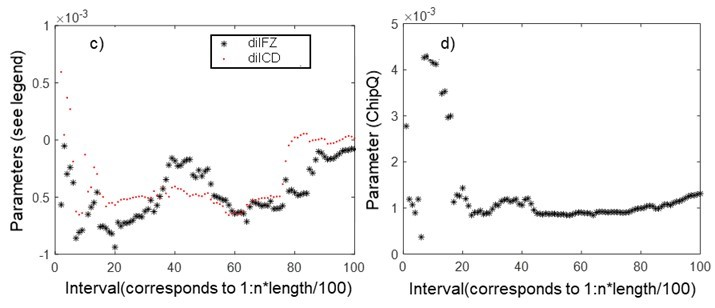Fig. 8. Model parameters (DC gains) for fiber length related to SpE (a), gapFZ (b), gapCD (b), dilFZ (c), dilCD (c), chipquality (d) versus a consecutively increased data set based on the entire set of data (800 h)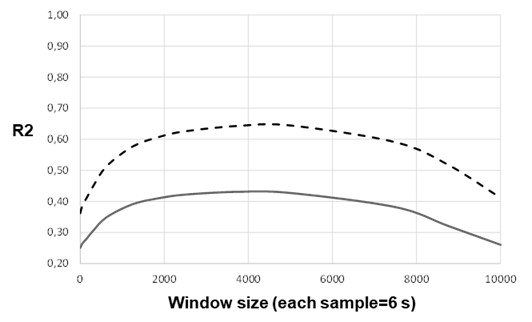Fig. 9. The coefficient of determination for the estimated shives(wide) using three different sets of predictors versus a varying window size. Solid: ux = [SpE, dilFZ, dilCD, gapFZ, gapCD, chipquality]. Dached: ux = [SpE, dilFZ, dilCD, Tinit, TmaxFZ, TCD (per), gapFZ, gapCD, chipquality]

Model Validation

It is important to choose the size of the time series in a proper way to cover enough process changes in all predictors. In this paper, a 1400-h validation set was studied.

To interpret the validation of the responses, a window size of 4000 samples (i.e., about 7 h) was applied both for shives(wide) and fiber length to describe the uncertainties in the pulp property measurements.

In Figs. 10 and 11, where the models described in Table 2 were visualized before and after filtering, it is clear that most of the dynamics could be captured. It is also interesting to see in Figs. 10 and 11 that the original models derived from the training set (Case A and Case F) seemed to follow the filtered measurements quite well. Note, when visualizing the response in shives(wide), the predictors used were based on the external variables, Fig. 10, while the predictors were extended to comprise both external and internal variables when estimating fiber length (see Fig. 11).

It is obvious when studying the figures that all dynamics were not covered by the models. This indicates that the number of process variables in the prediction vector should be increased further. This will be analyzed further in the Outlook section below.